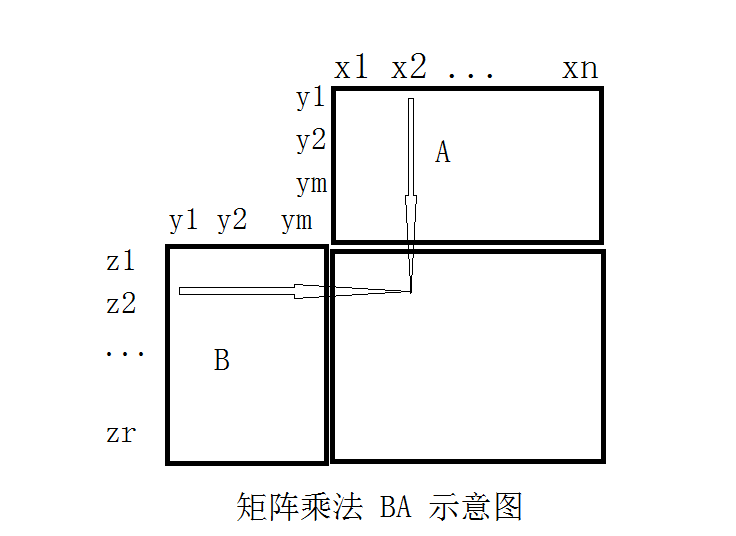1 2 3 4 »

# 再谈矩阵与矩阵乘法

《图示矩阵分块乘法》《理解矩阵与矩阵乘积（三）》《理解矩阵与矩阵乘积（二）》《理解矩阵与矩阵乘积（一）》

1                     a11          a12         a13                       a1n
2                     a21          a22         a23                       a2n
…                     …..           ….          ….                         ….
m                    am1         am2         am3                     ann

1                       ?
2

r

c11=a11b11+a21b12+…+am1b1m。# 图示矩阵分块乘法# Linear Algebra Done Right第十章注记和部分习题

L\inear Algebra Done Right 还有一章总结没有写，现在我的主要数学精力已经转向了另一个更为有趣的方向：复分析，读的是早已经买回的书《复分析，可视化方法》。这是一本读来让人心潮澎湃的书，曾经读了这本书的第一章，开设这个博客很大一部分原因还是想写写读这本书时的一些感受和记录它当中的问题。但三年多没有碰过数学我的数学能力已经回退到最原始状态了。它的译后记中说需要读者”比较认真地读过微积分和线性代数”，可是不光线性代数方面本来当初学得就不扎实，就连几年前十分拿手的数学分析我也基本上忘光了（当然初等微积分还记得一些）。我想务必要让自己的数学能力恢复一下再来读这本书，所以后来就读了《L\inear Algebra Done Right》并作了这些札记。

1 一个线性变换可以表示成 $$ST-TS$$ （其中 $$S$$ 与 $$T$$ 也是线性变换）的充要条件

http://tieba.baidu.com/f?kz=847919631

$\begin{pmatrix}a&b \\ c&d\end{pmatrix}$ 如果 $$a,d$$ 其一为 0 则命题得证。故以下假设 $$ad\not=0$$。

$\begin{pmatrix}1&k \\ 0&1\end{pmatrix}\begin{pmatrix}a&0 \\ 0&d\end{pmatrix}\begin{pmatrix}1&-k \\ 0&1\end{pmatrix}=\begin{pmatrix}a&kd-ka \\ 0&d\end{pmatrix}$ 取 $$k\not=0$$ 即可把右上角元素变为非零。故以下假设 $$b\not=0$$。那么考虑以下相似变换
$\begin{pmatrix}1&0 \\ -k&1\end{pmatrix}\begin{pmatrix}a&b \\ c&d\end{pmatrix}\begin{pmatrix}1&0 \\ k&1\end{pmatrix}=\begin{pmatrix}a+kb&b \\ c-ka+kd-k^2b&d-kb\end{pmatrix}$ 由于 $$b\not=0$$，那么我们总能取到适当的 $$k$$ 使得 $$a+kb=0$$ 或 $$c-kb=0$$。证毕。

8 设 $$V$$ 是内积空间且 $$v,w\in V$$。定义 $$T\in\mathcal L(V)$$ 为 $$Tu=\langle u,v\rangle w$$，找到 $$\mathrm{trace}\,T$$ 的表达式。

$\mathrm{trace}\,T=\sum_{i=1}^n\langle Te_i,e_i\rangle$ 设 $$e_1=w/|w|$$，将其扩充成标准正交基底 $$e_1,\dots,e_n$$，那么
$\mathrm{trace}\,T=\sum_{i=1}^n\langle Te_i,e_i\rangle=\langle w,v\rangle$ 如果 $$w=0$$ 则显然这个表达式也成立。

$\mathrm{trace}\,T=\langle Te_1,e_1\rangle=\langle w,v\rangle$。

9 证明如果 $$P\in\mathcal L(V)$$ 满足 $$P^2=P$$，那么 $$\mathrm{trace}\,P$$ 是非负整数。

15 设 $$T\in\mathcal L(V)$$，证明如果对任意 $$S\in\mathcal L(V)$$ 都有 $$\mathrm{trace}\,ST=0$$，那么 $$T=0$$。

$$\mathrm{trace}\,ST=\mathrm{trace}\,A^HA=\sum |a_{ij}|^2$$，由题设条件有 $$a_{ij}=0$$，故 $$T=0$$。

（16题可以用15题的方法，也可用8题解答中用到的事实。17题应用16题结论，注意不等式右边等于 $$\mathrm{trace}\,T^*T$$，当选用标准正交基底使得 $$T$$ 对应上三角矩阵时，可看出左边是小于等于 $$|Te_1|^2+\dots+|Te_n|^2$$ 的。19题也可用16题的结论，并利用 $$\mathrm{trace}\,T^*T=\mathrm{trace}\,TT^*$$，证得 $$|T^*e_i|=|Te_i|$$，注意这是对任意标准正交基底都成立的，也就是把任意一个非零向量标准化并扩充成标准正交基底即可得 $$|T^*v|=|Tv|$$。）

18 设 $$V$$ 是内积空间，证明 $$\langle S,T\rangle=\mathrm{trace}\,(ST^*)$$ 定义了 $$\mathcal L(V)$$ 上的内积。

$$\langle S,T\rangle=\mathrm{trace}\,(ST^*)=\overline{\mathrm{trace}\,(TS^*)}=\overline{\langle T,S\rangle}$$

# Linear Algebra Done Right第九章注记和部分习题

1 定理 9.9 的另一种证明

$$p(A_1),\dots,p(A_m)$$

3 设 $$A$$ 是分块对角矩阵 $A=\begin{pmatrix}A_1&& 0 \\ &\ddots& \\ 0&&A_m\end{pmatrix}$ 其中 $$A_j$$ 为方阵，证明 $$A$$ 的特征值的集合等于 $$A_1,\dots,A_m$$ 特征值集合的并集。
4 设 $$A$$ 是分块对角矩阵$A=\begin{pmatrix}A_1&&* \\ &\ddots& \\ 0&&A_m\end{pmatrix}$

8 证明不存在 $$T\in\mathcal L(\mathbf R^7)$$ 使得 $$T^2+T+I$$ 是幂零变换。

（10题可利用8题的结论，11题利用10题的结论）

14 设 $$V$$ 是 2 维向量空间而 $$T\in\mathcal L(V)$$，证明如果
$$\begin{pmatrix}a&b \\ c&d\end{pmatrix}$$

# Linear Algebra Done Right第八章注记和部分习题

1 $$\dim\mathrm{null}\,T^k$$ 随着 k 的增加量

$$\dim\mathrm{null}\,T^k$$ 是 k 的单调递增函数，而且当 k 达到某一个数值之后，$$\dim\mathrm{null}\,T^k$$ 就恒定不变了。但是书上并没有说它的增加有何规律，是每次都增加相同的维数，还是增加的维数可能有变化？换句话说，$$\dim\mathrm{null}\,T^{k+1}-\dim\mathrm{null}\,T^k$$ 有什么规律？它是恒定不变的，还是忽大忽小的，或是有什么别的规律没有？

3 引理 8.40 的另一种证明

a) $$(v_1,Nv_1,\dots,N^{m(v_1)}v1,\dots,v_k,Nv_k,\dots,N^{m(v_k)}v_k)$$ 是 $$V$$ 的基底；
b) $$(N^{m(v_1)}v1,\dots,N^{m(v_k)}v_k)$$ 是 $$\mathrm{null}\,N$$ 的基底。

3 设 $$T\in\mathcal L(V)$$，m 是正整数，且 $$v\in V$$ 是满足 $$T^{m-1}v\not=0$$ 但 $$T^mv=0$$ 的向量。证明 $$(v, Tv, T^2v,\dots,T^{m-1}v)$$ 线性无关。

5 设 $$S,T\in\mathcal L(V)$$。证明如果 $$ST$$ 是幂零的，那么 $$TS$$ 也是幂零的。

13 设 $$V$$ 是 n 维复向量空间，且 $$T\in\mathcal L(V)$$ 满足 $$\mathrm{null}\,T^{n-2}\not=\mathrm{null}\,T^{n-1}$$。证明 $$T$$ 至多有两个不同的特征值。

20 设 $$T\in\mathcal L(V)$$ 是可逆变换。证明存在多项式 $$p\in\mathcal P(F)$$ 使得 $$T^{-1}=p(T)$$。

28 设 $$a_0,\dots,a_{n-1}\in\mathbf C$$，某线性变换在标准基底下的矩阵是 $\begin{pmatrix}0&&&&&-a_0 \\ 1&0&&&&-a_1 \\ &1&\ddots&&&-a_2 \\ &&\ddots&&&\vdots \\ &&&&0&-a_{n-2} \\ &&&&1&-a_{n-1}\end{pmatrix}$ 找到它的最小多项式与特征多项式。

30 设 V 是复向量空间，且 $$T\in\mathcal L(V)$$。证明 $$V$$ 不能分解为 $$T$$ 的非平凡不变子空间的直和当且仅当 $$T$$ 的最小多项式形如 $$(T-\lambda I)^{\dim V}$$，其中 $$\lambda\in\mathbf C$$。